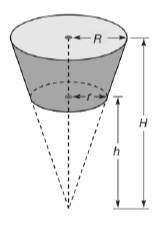Chapter 9.3, Problem 43E### Elementary Geometry for College St...

6th Edition
Daniel C. Alexander + 1 other
ISBN: 9781285195698

#### Solutions

Chapter
Section### Elementary Geometry for College St...

6th Edition
Daniel C. Alexander + 1 other
ISBN: 9781285195698
Textbook Problem
1 views

# A frustum of a cone is the portion of the cone bounded between the circular base and a plane parallel to the base. With dimensions are indicated, show that the volume of the frustum of the cone isV = 1 3 π R 2 H − 1 3 π r h 2

To determine

To show:

The volume of the frustum of the cone is V=13πR2H13πr2h.

Explanation

Given:

A frustrum of a cone is the portion of the cone bounded between the circular base and a plane parallel to the base.

Formula used:

The volume of the circular cone is V=13πr2h.

Calculation:

The frustum of the cone is constructed by cutting a smaller circular cone away from the larger cone.

Let R and H be the radius and height of the larger cone.

Then its volume is V1=13πR2H.

Let r and h be the radius and height of the smaller cone.

Then its volume is V2=13πr2h

### Still sussing out bartleby?

Check out a sample textbook solution.

See a sample solution

#### The Solution to Your Study Problems

Bartleby provides explanations to thousands of textbook problems written by our experts, many with advanced degrees!

Get Started

#### In Exercise 11-14, factor the expression. x2+5x+6

Calculus: An Applied Approach (MindTap Course List)

#### Find the mean for the following set of scores: 2, 7, 9, 4, 5, 3, 0, 6

Essentials of Statistics for The Behavioral Sciences (MindTap Course List)

#### Multiply: 70601300

Elementary Technical Mathematics

#### let f(x) = x3 + 5, g(x) = x2 2, and h(x)= 2x + 4. Find the rule for each function. 3. fg

Applied Calculus for the Managerial, Life, and Social Sciences: A Brief Approach

#### Factor completely. x2y2

Trigonometry (MindTap Course List)

#### True or False: The graph at the right is the graph of a function.

Study Guide for Stewart's Single Variable Calculus: Early Transcendentals, 8th